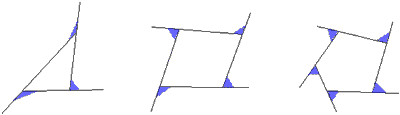# Sum of Exterior Angles

What is the sum of the exterior angles in a triangle? in a quadrilateral? in a pentagon? What is true about the sum of the exterior angles in any convex polygon? Why?